# Discussion Forum

Discussion Closed This discussion was created more than 6 months ago and has been closed. To start a new discussion with a link back to this one, click here.

## Maxwell stress, fluid volume force, axisymmetric

The below post is related to an archived discussion

So I have seen variations of this question around, like in the post above, but I still cannot make out the right answer for my problem.

I am simulating two-phase flow in an electrostatic field in a 2D axisimmetric domain. The two fluids have a different permittivity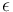, so that dielectrophoretic body forces are expected. I hoped that there was a ready made Multiphysics coupling for that, but there seems to be none in 5.4 + my set of modules.

Ok, modelling then. In the laminar flow physics tree, I add a volume force node. My understanding is that the force is given by the divergence of the stress tensor, as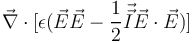. In cylindrical coordinates, that amounts to, for the two components r, z (e.g. wikipedia):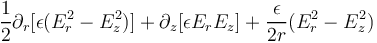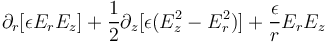The question is how to write this force term inside Comsol. I'm confused among three possibilities:

1. like written above, translated in code:
``````epsilon0_const*( d(es.epsilonr_iso*(es.Er^2-es.Ez^2)/2,r) + d(es.epsilonr_iso*es.Er*es.Ez,z) + es.epsilonr_iso*(es.Er^2-es.Ez^2)/(2*r) )
epsilon0_const*( d(es.epsilonr_iso*es.Er*es.Ez,r) + d(es.epsilonr_iso*(es.Ez^2-es.Er^2)/2,z) + es.epsilonr_iso*es.Er*es.Ez/r) )
``````

but this would be singular at the axis;

1. removing the 1/r terms, "because in physics interfaces Comsol takes care":
``````epsilon0_const*( d(es.epsilonr_iso*(es.Er^2-es.Ez^2)/2,r) + d(es.epsilonr_iso*es.Er*es.Ez,z) )
epsilon0_const*( d(es.epsilonr_iso*es.Er*es.Ez,r) + d(es.epsilonr_iso*(es.Ez^2-es.Er^2)/2,z) )
``````
1. Multiply 1. by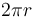, which removes the singularity, because an integration over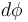is assumed:
``````pi*epsilon0_const*( r*d(es.epsilonr_iso*(es.Er^2-es.Ez^2),r) + 2*r*d(es.epsilonr_iso*es.Er*es.Ez,z) + es.epsilonr_iso*(es.Er^2-es.Ez^2) )
pi*epsilon0_const*( 2*r*d(es.epsilonr_iso*es.Er*es.Ez,r) + r*d(es.epsilonr_iso*(es.Ez^2-es.Er^2),z) + 2*es.epsilonr_iso*es.Er*es.Ez) )
``````

Anther possibility comes to mind, that is to include the diagonal contribution in the pressure and the nondiagonal contribution in the deviatoric tensor, but it doesn't seem available in the volume force interface.

5 Replies Last Post Oct 22, 2019, 5:13 AM EDT

Posted: 11 months ago
Updated: 11 months ago

For all those interested, for the time being, I resorted to use this form:

``````epsilon0_const*(d(es.epsilonr_iso*(es.Er^2-es.Ez^2)/2,r) + d(es.epsilonr_iso*es.Er*es.Ez,z) + es.epsilonr_iso*if(abs(r)<0.001*h_spatial,d(es.Er^2-es.Ez^2,r),(es.Er^2-es.Ez^2)/r)/2)
epsilon0_const*(d(es.epsilonr_iso*es.Er*es.Ez,r) + d(es.epsilonr_iso*(es.Ez^2-es.Er^2)/2,z) + es.epsilonr_iso*if(abs(r)<0.001*h_spatial,d(es.Er*es.Ez,r),es.Er*es.Ez/r))
``````

that is, #1 above, with a conditional `if` which switches the 1/r term to a derivative close to r=0. This is inspired by similar terms seen peeping inside the Equation Views of the tree.

Posted: 9 months ago
Updated: 9 months ago

Or instead to calculate EM force on the body, you can add electrical current node and add "force calculation node" it will automatically give you the exact electromagnetic force (Fx,Fy, Fz) for the selected domain.!

-------------------
RsG

Posted: 9 months ago
Updated: 9 months ago

Or instead to calculate EM force on the body, you can add electrical current node and add "force calculation node" it will automatically give you the exact electromagnetic force (Fx,Fy, Fz) for the selected domain.!

Is it a feature only on 5.4? How to add it? Cause I can't find just a node in my 5.3a. I also check the reference manual for 5.4 but can't find anything about 'Force Calculation'.

Posted: 9 months ago
Updated: 9 months ago

Hello "Physics student",

My guess is that you are not seeing the "Force Calculation" feature because of the modules that are included, and not included, on your license. Does your license include the AC/DC Module or the MEMS Module? That feature is available in those two products. You can check what modules are included on your license by going to File > Licensed and Used Products.

Best regards,

Jeff

-------------------
Jeff Hiller